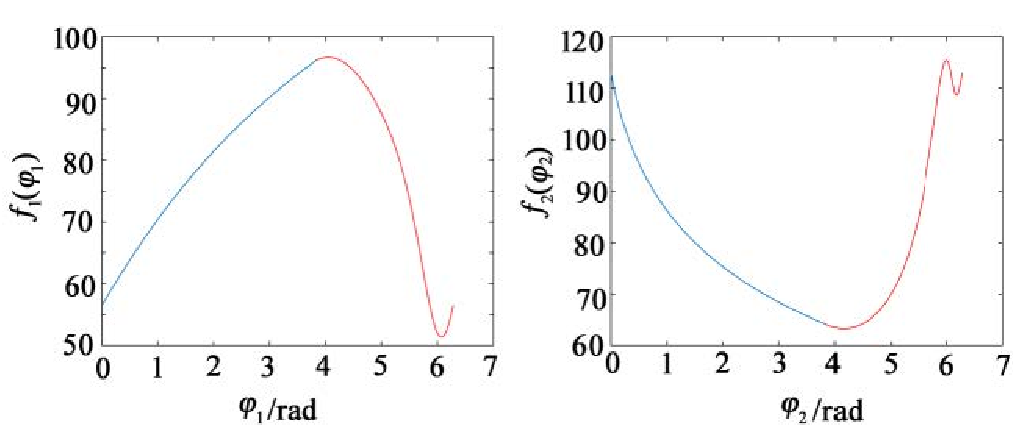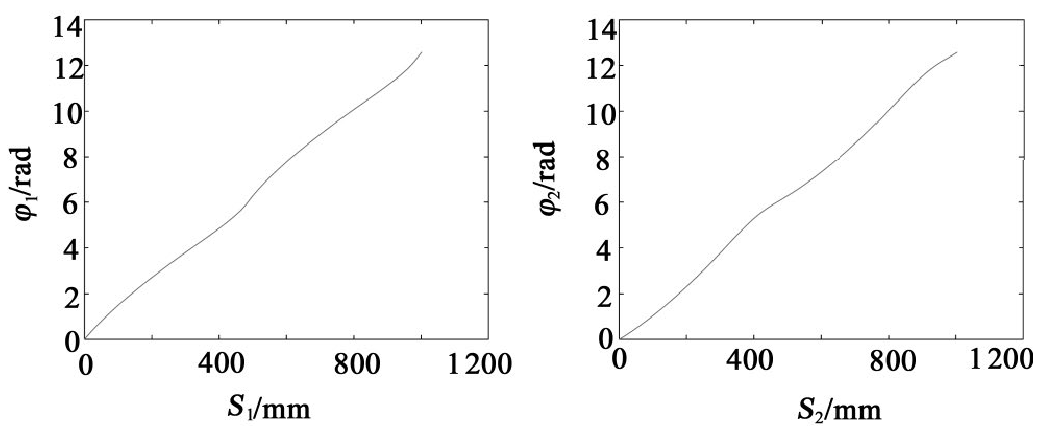# Solving arc length of pitch curve of noncircular gear

Suppose that the pitch curve arc lengths of the driving and driven noncircular gears are S1 and S2, respectively

The derivation of the formula shows that:

By substituting the known quantity into the formula, the integral function F1 is obtained（ φ 1) And F2（ φ 2) , and then draw φ 1- f1( φ 1) And φ 2-f2( φ 2) The relationship curve is shown in Figure 1-2.φ 1- f1( φ 1) Relationship curve φ 2-f2( φ 2) Relationship curve

The relationship curve in Figure 1-2 is numerically integrated by trapezoidal method to obtain the relationship curve as shown in Figure 3-4, and S1 = S2 = 500.299 mm is calculated. Due to the need of subsequent interpolation calculation, the y-axis coordinate range of figure 3-4 is expanded to [0,4] π] Rad, the corresponding X-axis coordinates also increase to twice the arc length of the pitch curve.S1- φ 1 relation curve S2- φ 2 Relationship curve